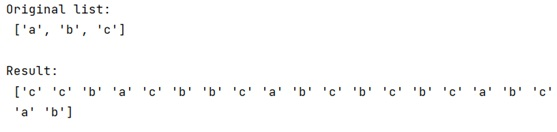# How to do weighted random sample of categories in Python?

In this tutorial, we will learn how to do weighted random sample of categories in Python? By Pranit Sharma Last updated : April 05, 2023

Suppose that we are given a list of tuples, where each tuple consists of a probability and an item and we need to sample an item according to its probability.

For example, if we are given the list as [ (.3, 'a'), (.4, 'b'), (.3, 'c')], we need to sample 'b' 40% of the time.

## Weighted random sample of categories

For weighted random sample of categories, we will extract the items from the list of tuples and separate the probabilities on one hand. To sample the items, we will apply numpy.random.choice() over the item array and pass a specific size also, pass the probability array.

Let us understand with the help of an example,

## Python code for weighted random sample of categories

```# Import numpy
import numpy as np

# Creating an item list
l = ['a','b','c']

# Display list
print("Original list:\n",l,"\n")

# Creating a probability list
p = [0.3,0.4,0.3]

# random sample of item
res = np.random.choice(l,size=20,p=p)

# Display result
print("Result:\n",res,"\n")
```

### OutputNote: We can see that 'b' occurs exactly 40% time in the sample array.